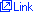(Last updated : 2021-03-31 13:43:55)
 Suzuki Toshiyuki    Kanagawa University  Faculty of Engineering Department of Mathematics    Assistant Professor
■ Academic conference presentation
 1 2017/10 Construction of wave operators for Hartree equations with a critical Hardy potential (Theory of Evolution Equation and Mathematical Analysis of Nonlinear Phenomena) 2 2014/07 Wellposedness for nonlinear Schrödinger equations with inverse-square potentials (The 10th AIMS Conference on Dynamical Systems, Differential Equations and Applications) 3 2013/08 Critical case of nonlinear Schrödinger equations with inverse-square potentials (Equadiff 13) 4 2012/10 Weak solvability for abstract nonlinear Schrödinger equations (New developments of the theory of evolution equations in the analysis of non-equilibria)
■ Book and Papers
 1. Papers Semilinear Schrödinger equations with a potential of some critical inverse-square type  (Single)  2020/062. Papers Scattering theory for semilinear Schrödinger equations with an inverse-square potential via energy methods  (Single)  2019/063. Papers Virial identities for nonlinear Schrödinger equations with a critical coefficient inverse- square potential  (Single)  2017/094. Papers Scattering theory for Hartree equations with inverse-square potentials  (Single)  2017/075. Papers Solvability of nonlinear Schrödinger equations with some critical singular potential via generalized Hardy-Rellich inequalities  (Single)  2016/045 display All display(15)
■ Belonging society
 1 2010/10～ Mathematical Society of Japan■ Present specialized field
 Key Word：Nonlinear partial differential equations, Schrödinger equations